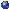## Events

##### Analysis Of Complex Physical SystemsMichael Hott, Zoom: On the emergence of a quantum Boltzmann equation in the presence of a Bose-Einstein condensate
Wednesday, September 29, 2021, 11:00am - 12:00pm
The mathematically rigorous derivation of a nonlinear Boltzmann equation from first principles is an extremely active research area. In classical physical systems, this has been achieved in various models, based on a variety of fundamental works. In the quantum case, the problem has essentially remained open. I will explain how a cubic quantum Boltzmann equation arises within the fluctuation dynamics of a Bose-Einstein condensate, starting with the von Neumann equation for an interacting Boson gas. This is based on joint work with Thomas Chen.Location: Zoom